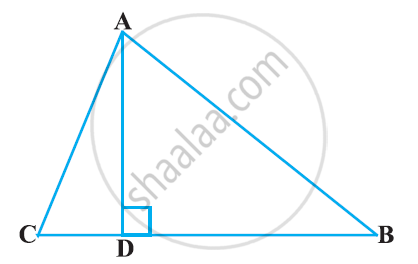# The perpendicular from A on side BC of a Δ ABC intersects BC at D such that DB = 3CD . Prove that 2AB^2 = 2AC^2 + BC^2. - Mathematics

The perpendicular from A on side BC of a Δ ABC intersects BC at D such that DB = 3CD . Prove that 2AB2 = 2AC2 + BC2.#### Solution

Applying Pythagoras theorem for ΔACD, we obtain

AD2 = AC2 - DC2  ... (1)

Applying Pythagoras theorem in ΔABD, we obtain

AD2 = AB2 - DB2 ...(2)

From equation 1 and 2 we obtain

AC2 - DC2 = AB2 - DB2 ....(3)

it is given that 3DC = DB

:.DC = (BC)/4

Putting these value in equation 3 we obtain

(AC)^2 - ((BC)/4) ^2 = (AB)^2 - ((3BC)/4)^2

(AC)^2 - (BC)^2/16 = (AB)^2-

16AC2 - BC2 = 16AB2 - 9BC2

16AB2 - 16AC2  8BC2

2AB2 = 2AC2 + BC2

Concept: Right-angled Triangles and Pythagoras Property
Is there an error in this question or solution?

#### APPEARS IN

NCERT Class 10 Maths
Chapter 6 Triangles
Exercise 6.5 | Q 14 | Page 151